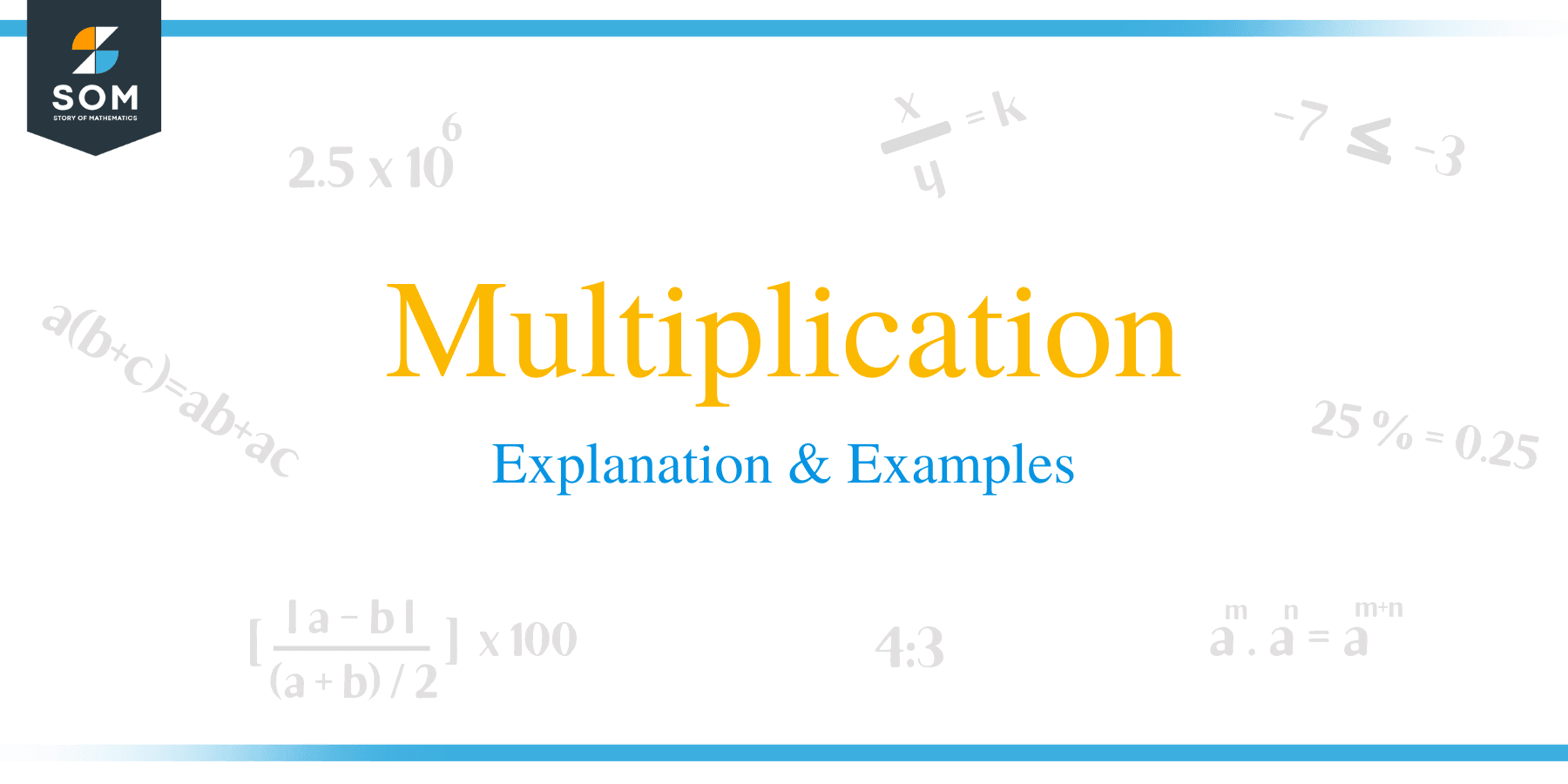# Multiplication – Explanation & Examples

##What is Multiplication?

Multiplication is a mathematical operation of finding the result of two or more numbers by repeated numbers’ additions.

Multiplication is usually denoted by a cross sign (x). However, other symbols such as an asterisk (*), dot(.), and the phrase “times” are also used.

## Parts of Multiplication

A multiplication sentence consists of two parts, namely the mathematical expression and the product. A mathematical expression consists of factors and the operator or symbol of multiplication.

For example, in a mathematical expression: 5 x 2 = 10, the portion “2 x 5” is the mathematical expression composed of 2 and 5 as factors and operators. The product, in this case, is 10.

We can further breakdown factors into multiplicand and multiplier:

• A multiplicand is a number multiplied by another number.
• A multiplier is a number with which to multiply.
• Product is a result of multiplying.## Properties of Multiplication

Learning properties of multiplication helps simplify and solve mathematical problems involving multiplication.

• Commutative

In multiplication, the commutative property implies that the multiplication of two or more numbers does not affect the final answer. In general, for a multiplication sentence: m x n = n x m. For example, 4 x 5 is the same as 5 x 4. This property also applies when multiplying a large group of numbers. For instance, 4 x 3 x 2 = 2 x 3 x 4.

• Associative

In multiplication, the associative property states that grouping of the numbers does not affect the ultimate answer when multiplying a series of numbers together. Generally, groupings in any mathematical expression are indicated by parentheses or brackets. We can summary this property as: m x (n x p) = (m x n) x p. For example, (2 x 4) x 6 = 2 x (4 x 6).

• Identity

This property states that the multiplication of any number with number one does not change its value. In other words, this property can be written as 1 x a = a. For example, 1 x 8 = 8.

• Distributive

The distributive property for multiplication states that an expression consisting of the addition or subtraction of values multiplied by a number is equivalent to the sum or difference of the expression numbers.

In general, m x (n + p) = m x n + m x p, and m x (n – p) = m x n – m x p. For example, 2 x (3 + 4) = 2 x 3 + 2 x 4.## Multiplication Chart

An array is a grid/multiplication chart consisting of numbers in rows and columns. Multiplication using a multiplication chart is easier because the product between two numbers is found by counting the number of rows and multiplying by the corresponding number of columns.

For example, in a grip consisting of 9 in the column and 6 in the row, the product in the grid is 54.

 x 1 2 3 4 5 6 7 8 9 10 11 12 1 1 2 3 4 5 6 7 8 9 10 11 12 2 2 4 6 8 10 12 14 16 18 20 22 24 3 3 6 9 12 15 18 21 24 27 30 33 36 4 4 8 12 16 20 24 28 32 36 40 44 48 5 5 10 15 20 25 30 35 40 45 50 55 60 6 6 12 18 24 30 36 42 48 54 60 66 72 7 7 14 21 28 35 42 49 56 63 70 77 84 8 8 16 24 32 40 48 56 64 72 80 88 96 9 9 18 27 36 45 54 63 72 81 90 99 108 10 10 20 30 40 50 60 70 80 90 100 110 120 11 11 22 33 44 55 66 77 88 99 110 121 132 12 12 24 36 48 60 72 84 96 108 120 132 144

## How to Create Multiplication Sentences?

Learning how to create multiplication sentences is an essential skill to learners as it prepares them how to use mathematics practically. A student who knows how to create his/her own multiplication sentences can look at a four-by-four grid of numbers and will definitely tell that the grid consists of 16 items.

### How to Create a Sentence from a Word Problem

Creating multiplication sentences seems to throw students off. However, by reading and understanding this article, solving word problems should be easier for the students.

For instance, suppose Mary collected a basket of oranges if she has enough oranges to place 15 oranges per 3 rows. How many oranges did she collect? In this example, the multiplication sentence can be written as 15 x 3 = 45. Therefore, Mary collected 45 oranges.

### Practice Questions

1. A file has $24$ folders, and each folder contains $56$ sheets of paper. Which of the following shows the total sheets of papers in the file?

2. A carton of biscuits contains $24$ packets, and each packet contains $12$ biscuits. How many biscuits can be packed in $45$ cartons?

3. The cost of a textbook is $\$ 67$. How much would$102$textbooks cost? 4. A man has a monthly salary of$\$2625$. How much does he earn per year?

5. The cost of a chair and table are $\$ 452$and$\$1750$, respectively. What is the total cost of $15$ chairs and $30$ tables?